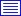# Partners

 Ewa Majchrzak

Recent publications
1.  Dziatkiewicz J., Kuś W., Majchrzak E., Burczyński T., Turchan Ł., Bioinspired Identification of Parameters in Microscale Heat Transfer, INTERNATIONAL JOURNAL FOR MULTISCALE COMPUTATIONAL ENGINEERING, ISSN: 1543-1649, DOI: 10.1615/IntJMultCompEng.2014007963, Vol.12, No.1, pp.79-89, 2014Abstract:
The paper is devoted to the identification of microscale heat-transfer parameters. The numerical modeling of short-pulse laser interaction with thin metal films is considered. The hyperbolic two-temperature model describing the temporal and spatial evolution of the lattice and electrons temperatures in the irradiated metal is applied. This model consists of four equations: two equations concern the electron and lattice temperatures; the later ones determine the dependencies between heat fluxes and temperatures. The short-pulse laser interaction with the film is taken into account by introducing an internal volumetric heat source to the equation describing the electron temperature. The equations concerning the electrons and lattice temperatures are joined by coupling factor G, which characterizes the energy exchange between phonons and electrons. The relations between electron heat flux and electron temperature and between the lattice heat flux and lattice temperature contain the parameters ?e and ?l, respectively. The parameter ?e is the relaxation time of free electrons in metals; the parameter ?l is the relaxation time in phonon collisions. The one-dimensional problem is analyzed. (Heat transfer in the direction perpendicular to the thin film is taken into account.) The nonflux conditions can be accepted at the front surface irradiated by a laser pulse and the back surface. The initial conditions are also assumed. The direct problem is solved by the explicit scheme of the finite difference method. The results of the computations are partially compared with the experimental data available in literature. The inverse problem discussed here consists in the simultaneous identification of three parameters, namely, the coupling factor G and relaxation times ?e and ?l. To solve such a problem, the electron temperature history at the irradiated surface of the thin film is taken into account. The inverse problems can be formulated as optimization problems and solved by means of bioinspired algorithms. The objective function is formulated on the basis of the known measured and numerical simulated values of temperature. The minimization of the objective function allows one to find the design variables vector, which may contain the parameters of the coupling factor and time coefficients in the presented case. The inverse problems are ill-defined problems, and the identification may lead to different results with the same objective function value. The objective function can have many local minima, and therefore the bioinspired algorithm is used in the paper.

Keywords:
multiscale modeling, two-temperature model, identification, bioinspired algorithms

Affiliations:
 Dziatkiewicz J. - Silesian University of Technology (PL) Kuś W. - Silesian University of Technology (PL) Majchrzak E. - other affiliation Burczyński T. - other affiliation Turchan Ł. - Silesian University of Technology (PL)### IPPT PANPawińskiego 5B, 02-106 Warsaw +48 22 826 12 81 (central) +48 22 826 98 15